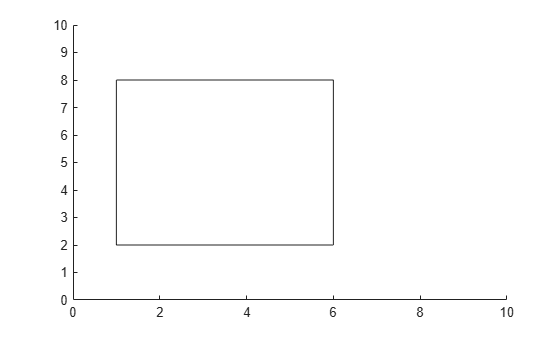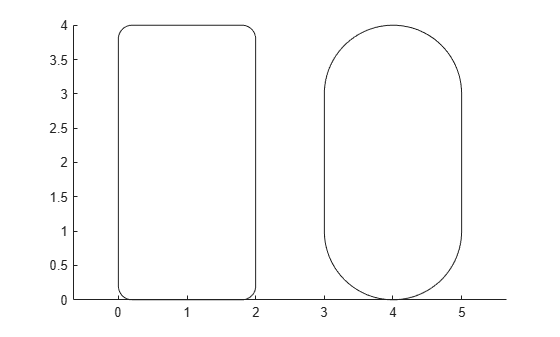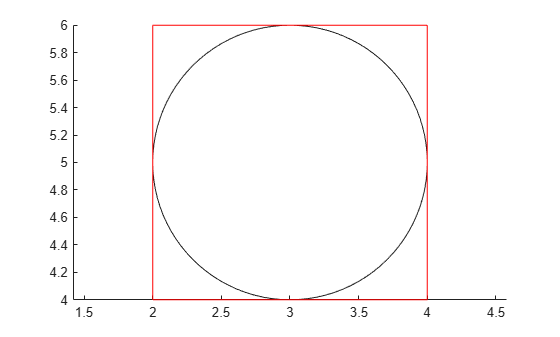# rectangle

Create rectangle with sharp or curved corners

## Syntax

``rectangle('Position',pos)``
``rectangle('Position',pos,'Curvature',cur)``
``rectangle(___,Name,Value)``
``rectangle(ax,___)``
``r = rectangle(___)``

## Description

example

````rectangle('Position',pos)` creates a rectangle in 2-D coordinates. Specify `pos` as a four-element vector of the form ```[x y w h]``` in data units. The `x` and `y` elements determine the location and the `w` and `h` elements determine the size. The function plots into the current axes without clearing existing content from the axes.```

example

````rectangle('Position',pos,'Curvature',cur)` adds curvature to the sides of the rectangle. For different curvatures along the horizontal and vertical sides, specify `cur` as a two-element vector of the form `[horizontal vertical]`. For the same length of curvature along all sides, specify `cur` as a scalar value. Specify values between 0 (no curvature) and 1 (maximum curvature). Use `[1 1]` to create an ellipse or circle.```

example

````rectangle(___,Name,Value)` specifies rectangle properties using one or more name-value pair arguments. For example, `'FaceColor','red'` specifies a red fill color. You can specify rectangle properties with any of the input argument combinations in the previous syntaxes.```
````rectangle(ax,___)` creates the rectangle in the axes specified by `ax` instead of in the current axes (`gca`). The option `ax` can precede any of the input argument combinations in the previous syntaxes.```

example

````r = rectangle(___)` returns the rectangle object. Use `r` to modify properties of the rectangle after it is created. For a list of properties, see Rectangle Properties. You can return an output argument with any of the previous syntaxes. ```

## Examples

collapse all

Draw a rectangle that has a lower left corner at the point (1,2). Make the width of the rectangle 5 units and the height 6 units. Then, change the axis limits.

```rectangle('Position',[1 2 5 6]) axis([0 10 0 10])```Draw a rectangle that has a lower left corner at (0,0) and an upper right corner at (2,4). Create the rectangle with curved corners by specifying the curvature as the scalar value `0.2`. For data units of equal length along both the x-axis and y-axis, use `axis equal`.

```figure rectangle('Position',[0 0 2 4],'Curvature',0.2) axis equal```Add a second rectangle that has the shortest side completely curved by specifying the curvature as the scalar value `1`.

`rectangle('Position',[3 0 2 4],'Curvature',1)`Add a third rectangle and use different curvatures along the horizontal and vertical sides. Use a horizontal curvature of 0.5 to make 50 percent of each horizontal side curved. Use a vertical curvature of 1 to make both vertical sides completely curved.

`rectangle('Position',[6 0 2 4],'Curvature',[0.5,1])`Draw a circle by setting the `Curvature` property to `[1 1]`. Draw the circle so that it fills the rectangular area between the points (2,4) and (4,6). The `Position` property defines the smallest rectangle that contains the circle.

```pos = [2 4 2 2]; rectangle('Position',pos,'Curvature',[1 1]) axis equal```Draw a red rectangle using the same position values to show how the circle fills the rectangular area.

`rectangle('Position',pos,'EdgeColor','r')`Draw a rectangle and specify the fill color, outline color, and outline width.

```rectangle('Position',[1,2,5,10],'FaceColor',[0 .5 .5],'EdgeColor','b',... 'LineWidth',3)```Add a rectangle to the current axes and return the rectangle object, `r`.

`r = rectangle('Position',[0 0 1 1]')````r = Rectangle with properties: FaceColor: 'none' EdgeColor: [0 0 0] LineWidth: 0.5000 LineStyle: '-' Curvature: [0 0] Position: [0 0 1 1] Show all properties ```

Modify the rectangle after creation using `r`. Change the fill color, outline color, and outline width.

```r.FaceColor = [0 .5 .5]; r.EdgeColor = 'b'; r.LineWidth = 3;```## Input Arguments

collapse all

Size and location of the rectangle, specified as a four-element vector of the form `[x y w h]`. The `x` and `y` elements define the coordinate for the lower left corner of the rectangle. The `w` and `h` elements define the dimensions of the rectangle.

All values are in data units.

Example: `rectangle('Position',[0.5 0.5 0.3 0.4])`

Data Types: `double` | `single` | `int8` | `int16` | `int32` | `int64` | `uint8` | `uint16` | `uint32` | `uint64`

Amount of horizontal and vertical curvature, specified as a two-element vector or a scalar value. Use this property to vary the shape of the rectangle from rectangular to ellipsoidal.

The horizontal curvature is the fraction of the width that is curved along the top and bottom edges. The vertical curvature is the fraction of the height that is curved along the left and right edges.

• To use different horizontal and vertical curvatures, specify a two-element vector of the form ```[horizontal vertical]```. The `horizontal` element determines the horizontal curvature and the `vertical` element determines the vertical curvature. Specify the values between 0 (no curvature) and 1 (maximum curvature). For example, a value of ```[0 0]``` creates a rectangle with square edges and value of ```[1 1]``` creates an ellipse.

• To use the same curvature for the horizontal and vertical edges, specify a scalar value between 0 and 1, inclusive. The shorter dimension determines the length of the curvature.

Example: `[0.5 0.6]`

Example: `0.75`

Data Types: `double`

Axes object. If you do not specify an axes object, then `rectangle` uses the current axes.

### Name-Value Arguments

Specify optional pairs of arguments as `Name1=Value1,...,NameN=ValueN`, where `Name` is the argument name and `Value` is the corresponding value. Name-value arguments must appear after other arguments, but the order of the pairs does not matter.

Before R2021a, use commas to separate each name and value, and enclose `Name` in quotes.

Example: ```rectangle('Position',[0 0 1 1],'EdgeColor','k','FaceColor',[0 .5 .5])```

Fill color, specified as an RGB triplet, a hexadecimal color code, a color name, or a short name.

For a custom color, specify an RGB triplet or a hexadecimal color code.

• An RGB triplet is a three-element row vector whose elements specify the intensities of the red, green, and blue components of the color. The intensities must be in the range `[0,1]`, for example, ```[0.4 0.6 0.7]```.

• A hexadecimal color code is a character vector or a string scalar that starts with a hash symbol (`#`) followed by three or six hexadecimal digits, which can range from `0` to `F`. The values are not case sensitive. Therefore, the color codes `"#FF8800"`, `"#ff8800"`, `"#F80"`, and `"#f80"` are equivalent.

Alternatively, you can specify some common colors by name. This table lists the named color options, the equivalent RGB triplets, and hexadecimal color codes.

Color NameShort NameRGB TripletHexadecimal Color CodeAppearance
`"red"``"r"``[1 0 0]``"#FF0000"``"green"``"g"``[0 1 0]``"#00FF00"``"blue"``"b"``[0 0 1]``"#0000FF"``"cyan"` `"c"``[0 1 1]``"#00FFFF"``"magenta"``"m"``[1 0 1]``"#FF00FF"``"yellow"``"y"``[1 1 0]``"#FFFF00"``"black"``"k"``[0 0 0]``"#000000"``"white"``"w"``[1 1 1]``"#FFFFFF"``"none"`Not applicableNot applicableNot applicableNo color

Here are the RGB triplets and hexadecimal color codes for the default colors MATLAB® uses in many types of plots.

RGB TripletHexadecimal Color CodeAppearance
`[0 0.4470 0.7410]``"#0072BD"``[0.8500 0.3250 0.0980]``"#D95319"``[0.9290 0.6940 0.1250]``"#EDB120"``[0.4940 0.1840 0.5560]``"#7E2F8E"``[0.4660 0.6740 0.1880]``"#77AC30"``[0.3010 0.7450 0.9330]``"#4DBEEE"``[0.6350 0.0780 0.1840]``"#A2142F"`Example: `'blue'`

Example: ```[0 0 1]```

Example: `'#0000FF'`

Outline color, specified as an RGB triplet, a hexadecimal color code, a color name, or a short name. The default RGB triplet value of `[0 0 0]` corresponds to black.

For a custom color, specify an RGB triplet or a hexadecimal color code.

• An RGB triplet is a three-element row vector whose elements specify the intensities of the red, green, and blue components of the color. The intensities must be in the range `[0,1]`, for example, ```[0.4 0.6 0.7]```.

• A hexadecimal color code is a character vector or a string scalar that starts with a hash symbol (`#`) followed by three or six hexadecimal digits, which can range from `0` to `F`. The values are not case sensitive. Therefore, the color codes `"#FF8800"`, `"#ff8800"`, `"#F80"`, and `"#f80"` are equivalent.

Alternatively, you can specify some common colors by name. This table lists the named color options, the equivalent RGB triplets, and hexadecimal color codes.

Color NameShort NameRGB TripletHexadecimal Color CodeAppearance
`"red"``"r"``[1 0 0]``"#FF0000"``"green"``"g"``[0 1 0]``"#00FF00"``"blue"``"b"``[0 0 1]``"#0000FF"``"cyan"` `"c"``[0 1 1]``"#00FFFF"``"magenta"``"m"``[1 0 1]``"#FF00FF"``"yellow"``"y"``[1 1 0]``"#FFFF00"``"black"``"k"``[0 0 0]``"#000000"``"white"``"w"``[1 1 1]``"#FFFFFF"``"none"`Not applicableNot applicableNot applicableNo color

Here are the RGB triplets and hexadecimal color codes for the default colors MATLAB uses in many types of plots.

RGB TripletHexadecimal Color CodeAppearance
`[0 0.4470 0.7410]``"#0072BD"``[0.8500 0.3250 0.0980]``"#D95319"``[0.9290 0.6940 0.1250]``"#EDB120"``[0.4940 0.1840 0.5560]``"#7E2F8E"``[0.4660 0.6740 0.1880]``"#77AC30"``[0.3010 0.7450 0.9330]``"#4DBEEE"``[0.6350 0.0780 0.1840]``"#A2142F"`Example: `'blue'`

Example: ```[0 0 1]```

Example: `'#0000FF'`

Line width, specified as a positive value in point units.

Example: `0.75`

Line style, specified as one of the options listed in this table.

Line StyleDescriptionResulting Line
`"-"`Solid line`"--"`Dashed line`":"`Dotted line`"-."`Dash-dotted line`"none"`No lineNo line

## Version History

Introduced before R2006a